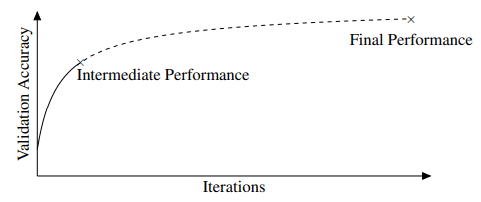# Catch up on the latest AI articles# How Much Learning Is Required To Compare Model Performance?

3 main points
✔️ Proposed a method to predict the final performance of the model during training
✔️ Compare and rank the final performance of the model using part of the learning curve
✔️ Accelerate your NAS up to 100 times faster

Learning to Rank Learning Curves
written by
Martin WistubaTejaswini Pedapati
(Submitted on 5 Jun 2020)

Subjects: Machine Learning (cs.LG); Computer Vision and Pattern Recognition (cs.CV); Machine Learning (stat.ML)## Introduction

In NAS (Network Architecture Exploration) and HPO (Hyperparameter Optimization), many different machine learning models are trained to discover the optimal structure and hyperparameters. The major challenge of these AutoMLs is that there is a large computational cost in actually training the models in the search process, such as model structure. Therefore, the establishment of a fast method for evaluating good and bad architectures is an important research topic in AutoML, including NAS.

In the paper presented in this article, a method was proposed to interrupt the learning of low performing architectures early to reduce the cost of learning. Experiments have demonstrated that NAS can be made up to 100 times faster with minimal performance loss.

Extrapolation of the learning curve is an existing method for predicting the final performance of a model at an early stage of training. As shown in the figure below, this method predicts the final performance of a model from the point when the learning curve is halfway obtained (the point of intermediate performance in the figure).Such an extrapolation method involves large computational costs since a large number of training curves are actually sampled. The method proposed in the paper, LCRankNet, does not predict the final performance of the model in this way.

To begin with, what is important in the NAS architecture search process is the relative performance of one model compared to another. There is no absolute performance requirement as to how well that model performs. Therefore, LCRankNet predicts the final performance of a model and directly predicts which model is better than the other, rather than comparing the superiority of a model based on its size.

### The term

The important aspects of the notation in the description of the method are discussed earlier.

In the following commentary, the entire learning curve (obtained when the learning is completed to the end) is denoted by $y_1,...,y_L,y_L$, where $y_i$ is the performance of the model (e.g. classification accuracy). In this case, $y_i$ represents the performance of the model (e.g. classification accuracy) and is determined at regular intervals (e.g. every few epochs).

The partial learning curve (obtained in the middle of learning) is also expressed as $y_1,...,y_l,y_l$, which is represented as $y_1,...,y_l$.